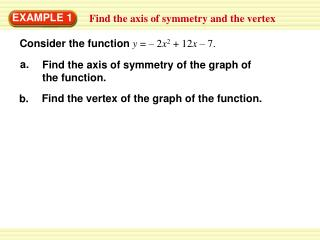Download PresentationEXAMPLE 1

# EXAMPLE 1 - PowerPoint PPT PresentationDownload Presentation## EXAMPLE 1

- - - - - - - - - - - - - - - - - - - - - - - - - - - E N D - - - - - - - - - - - - - - - - - - - - - - - - - - -
##### Presentation Transcript

1. a. Find the axis of symmetry of the graph of the function. Find the vertex of the graph of the function. b. EXAMPLE 1 Find the axis of symmetry and the vertex Consider the function y= – 2x2 + 12x – 7.

2. a. For the function y = –2x2 + 12x – 7, a = 2 and b = 12. x=– 12 b b = =3 2(– 2) 2a 2a b. The x-coordinate of the vertex is , or 3. – ANSWER The vertex is (3, 11). EXAMPLE 1 Find the axis of symmetry and the vertex SOLUTION Substitute – 2 for aand 12 for b. Then simplify. y = –2(3)2 + 12(3) – 7 = 11 Substitute 3 for x. Then simplify.

3. = = 1. 2(3) b –6 – x = – Find and draw the axis of symmetry: 2a EXAMPLE 2 Graphy = ax2 + bx + c Graphy = 3x2 – 6x + 2. STEP1 Determine whether the parabola opens up or down. Because a > 0, the parabola opens up. STEP 2 STEP 3 Find and plot the vertex.

4. The x-coordinate of the vertex isb , or1. – 2a EXAMPLE 2 Graph y = ax2 + b x + c To find the y-coordinate, substitute 1 for xin the function and simplify. y = 3(1)2– 6(1) + 2 =– 1 So, the vertex is(1, – 1). STEP 4 Plot two points. Choose two x-values less than the x-coordinate of the vertex. Then find the corresponding y-values.

5. EXAMPLE 2 Standardized Test Practice STEP5 Reflect the points plotted in Step 4 in the axis of symmetry. STEP6 Draw a parabola through the plotted points.

6. EXAMPLE 2 Graph y = ax2 + b x + c

7. 1. Find the axis of symmetry and vertex of the graph of the function y= x2 – 2x – 3. ANSWER ANSWER x = 1, (1, –4). for Examples 1 and 2 GUIDED PRACTICE 2. Graph the function y = 3x2 + 12x – 1. Label the vertex and axis of symmetry.